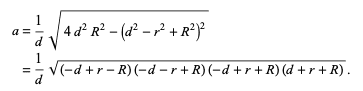# Expanding and simplifying brackets

• MHB
• Maggie_s2020

#### Maggie_s2020

Hello, I have been trying to solve the top line equation to get the result (the bottom line). I am searching for a clue (the steps) on how to obtain those four brackets as a result.Last edited by a moderator:

factoring the expression within the radical just involves the difference of squares ...

$4d^2R^2 - (d^2-r^2+R^2)^2$

$(2dR)^2 - (d^2-r^2+R^2)^2$

$[2dR -(d^2-r^2+R^2)] \cdot [2dR + (d^2-r^2+R^2)]$

$[-(d^2-2dR+R^2) + r^2] \cdot [(d^2+2dR+R^2) - r^2]$

$[r^2-(d-R)^2] \cdot [(d+R)^2 - r^2]$

$[(r-d+R)(r+d-R)] \cdot [(d+R-r)(d+R+r)]$

multiply the two middle factors by (-1) ...

$(-d+r+R)(-d-r+R)(-d+r-R)(d+r+R)$

Last edited by a moderator:
factoring the expression within the radical just involves the difference of squares ...

$4d^2R^2 - (d^2-r^2+R^2)$

$(2dR)^2 - (d^2-r^2+R^2)^2$

$[2dR -(d^2-r^2+R^2)] \cdot [2dR + (d^2-r^2+R^2)]$

$[-(d^2-2dR+R^2) + r^2] \cdot [(d^2+2dR+R^2) - r^2]$

$[r^2-(d-R)^2] \cdot [(d+R)^2 - r^2]$

$[(r-d+R)(r+d-R)] \cdot [(d+R-r)(d+R+r)]$

multiply the two middle factors by (-1) ...

$(-d+r+R)(-d-r+R)(-d+r-R)(d+r+R)$

THANK YOU SO SO SO MUCH! BLESS YOU!